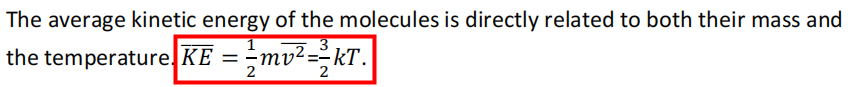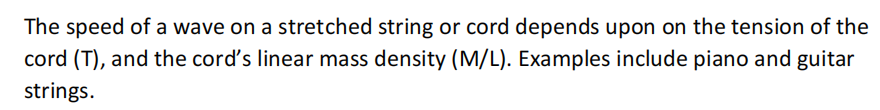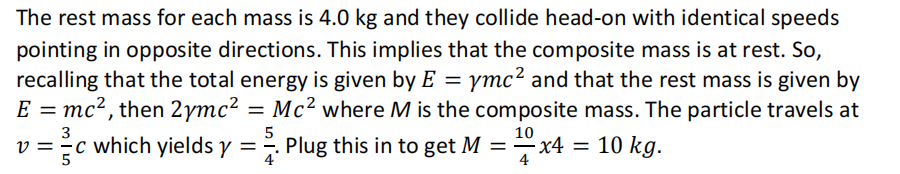# 2023物理碗竞赛报名中，内含历年真题和答案解析！

【1】In a mixture of hydrogen, oxygen, and nitrogen gases at room temperature, the molecules having the greatest average speed are those of

(A) Hydrogen (B) Oxygen (C) Nitrogen (D) All have the same speed (E) It depends upon the composition of the mixture【2】Consider a traveling wave on a string of length L, mass M, and tension T. A standing wave is set up. Which of the following is true?

(A) The wave velocity depends on M, L, T.
(B) The wavelength of the wave is proportional to the frequency.
(C) The velocity of a given particle in the string is equal to the wave velocity.
(D) The wavelength is proportional to T.
(E) The frequency depends upon L.【3】A lump of clay whose rest mass is 4.0 kg is traveling at three-fifths of the speed of light when it collides head-on with an identical lump going in the opposite direction at the same speed. If the two lumps stick together and no energy is radiated away, what is the mass of the composite lump?

(A) 4.0 kg (B) 6.4 kg (C) 8.0 kg (D) 10.0 kg (E) 13.3 kg【扫码联系老师领取报名表】